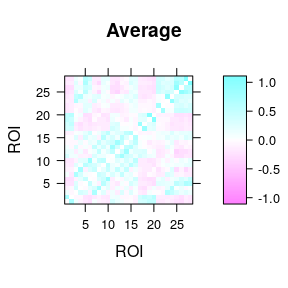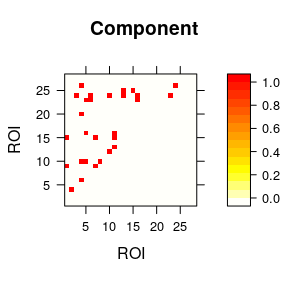# Network-Based R-statistics for linear models

This vignette documents the implementation of NBR 0.1.3 for linear models.

We will analyze the frontal3D dataset, which contains a 3D volume of 48 matrices, each matrix representing the functional connectivity between 28 nodes (in the frontal lobe). Phenotypic information (frontal_phen) includes diagnostic GROUP (patient or control), sex, and age. We will test for a GROUP effect.

library(NBR)
cmx <- NBR:::frontal3D          # Load 3D array
brain_labs <- NBR:::frontal_roi # Load node labels
phen <- NBR:::frontal_phen      # Load phenotypic info
dim(cmx)                        # Show 3D array dimensions
#>  28 28 48

We can plot the sample average matrix, with lattice::levelplot.

library(lattice)
avg_mx <- apply(cmx, 1:2, mean)
# Set max-absolute value in order to set a color range centered in zero.
flim <- max(abs(avg_mx)[is.finite(avg_mx)])
levelplot(avg_mx, main = "Average", ylab = "ROI", xlab = "ROI",
at = seq(-flim, flim, length.out = 100))As we can observe, this is a symmetric matrix with the pairwise connections of the 28 regions of interest (ROI) brain_labs. The next step is to check the phenotypic information (stored in phen) to perform statistic inferences edgewise. Before applying the NBR-LM, we check that the number of matrices (3rd dimension in the dataset) matches the number of observations in the phen data.frame.

head(phen)
#>     Group Sex   Age
#> 1 Control   F  8.52
#> 2 Control   M 16.16
#> 3 Patient   M 17.75
#> 4 Control   M 12.27
#> 5 Control   F 12.07
#> 6 Patient   F  8.71
nrow(phen)
#>  48
identical(nrow(phen), dim(cmx))
#>  TRUE

The data.frame contains the individual information for diagnostic group, sex, and chronological age. So, we are all set to perform an NBR-LM. We are going to test the effect of diagnostic group with a minimal number of permutations to check that we have no errors.

set.seed(18900217) # Because R. Fisher is my hero
before <- Sys.time()
nbr_group <- nbr_lm_aov(net = cmx, nnodes = 28, idata = phen,
mod = "~ Group", thrP = 0.01, nperm = 10)
#> Computing observed stats.
#> Computing permutated stats.
#> Permutation progress: ....
after <- Sys.time()
show(after-before)
#> Time difference of 6.696129 secs

Although ten permutations is quite low to obtain a proper null distribution, we can see that they take several seconds to be performed. So we suggest to parallelizing to multiple CPU cores with cores argument.

set.seed(18900217)
library(parallel)
before <- Sys.time()
nbr_group <- nbr_lm_aov(net = cmx, nnodes = 28, idata = phen,
mod = "~ Group", thrP = 0.01, nperm = 100, cores = detectCores())
after <- Sys.time()
length(nbr_group)

NBR functions return a nested list of at least two lists. The first list encompasses all the individual significant edges, their corresponding component and statistical inference (p < 0.01, in this example). In this case all the significant edges belong to a single component.

# Plot significant component
edge_mat <- array(0, dim(avg_mx))
edge_mat[nbr_group$components$Group[,2:3]] <- 1
levelplot(edge_mat, col.regions = rev(heat.colors(100)),
main = "Component", ylab = "ROI", xlab = "ROI")show(nbr_group$fwe$Group)
#>   Component ncomp ncompFWE     strn strnFWE
#> 1         1    28        0 64.52926       0

As we can observe, significant edges are displayed in the upper triangle of the matrix, and the second list (fwe) contains, for each term of the equation, the probability of the observed values to occur by chance, based on the null distribution.